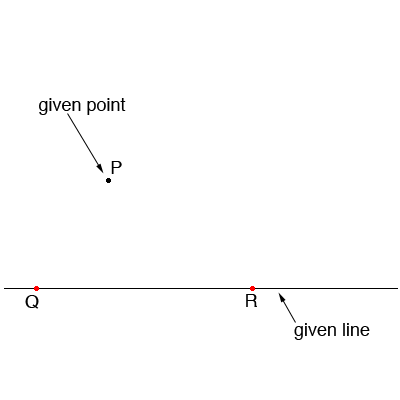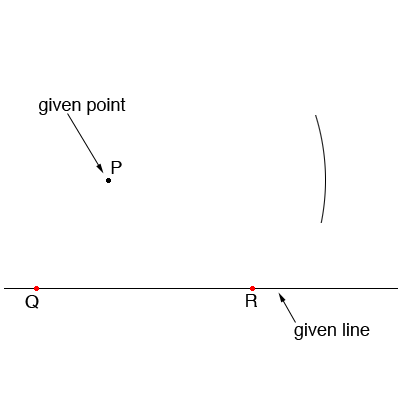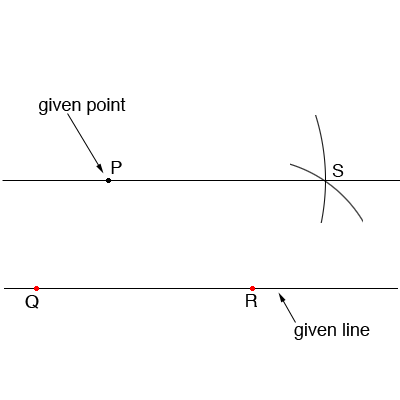SEARCH HOMEMath Central Quandaries & QueriesQuestion from john, a student: A parallel line to a given line through a given point not on the a given lineHi John,

Call the given point P. Mark two distinct points Q and R on the given line.Put the compass point at Q and the pencil point at R. Without changing the compass setting move the point to P and draw an arc.Put the compass point at Q and the pencil point at P. Without changing the compass setting move the point to R and draw an arc to intersect the previous arc at S. Draw a line containing P and S.Prove The line through P and S is parallel to the given line.

HarleyMath Central is supported by the University of Regina and The Pacific Institute for the Mathematical Sciences.